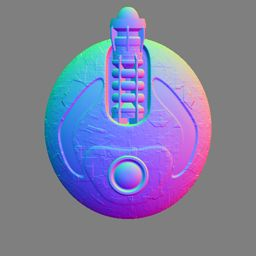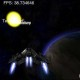Game Design, Programming and running a one-man games business…

# Stuck on a normal map thing…

My lack of maths skill will be my undoing…

I have some normal maps, you know the kinda thing…And I have all the code (dot product stuff) that plugs in the float3 that is the light direction, and then gives me a tint I can apply to the final pixel to get a nice pseudo 3D bump map effect. Thats all lovely and works, and is cool. The problem is, when I want to rotate that bump map, it obviously all turns to crap :D

This is NOT a case where I can just liue about the light angle, I already get all that… What I need (for this pre-processing cleverness) is a way of taking that image above, and effectively rotating the whole thing by a given angle, and working out what all the pixel colors would be in that case. This is an arbitrary rotating value (so not just 90 degrees or whatever). I’ll work it out eventually, but I suck at this, and I bet it’s easy. This isn’t speed-dependent stuff so slow is fine, either pure C++ maths or some shader code.

My current shader code for rendering using the normal map:

float4 normalcolor = tex2Dbias(g_samNormalMapColor, texCoord);
normalcolor.b = 0; //experiment to force only r or g channels

//convert it to +/- 1.0 range
normalcolor *= 2.0f;
normalcolor -= 1.0f;

float3 LightDirection;
LightDirection.x = cos(sprite_angle);
LightDirection.y = sin(sprite_angle);
LightDirection.z = 0;

float dot_prod = dot(LightDirection, normalcolor);

Thats just lovely, and obvioously I could lie about the angle I put in, but then how do I get the red and green out of it?

## 6 thoughts on Stuck on a normal map thing…

1.Nils says:

Just use a 2D rotation matrix and transform your normals with it. http://en.wikipedia.org/wiki/Rotation_matrix
It should be quite easy ;).

2.Nils says:

And here you go for some code sample:

float2x2 matRotation; //I may mixed up rows and columns here!? This is how it would be in GLSL…
matRotation = cos(angle);
matRotation = -sin(angle);
matRotation = sin(angle);
matRotation = cos(angle);

float3 normal = tex2Dbias(g_samNormalMapColor, texCoord)*2.0 – 1.0;

//The rotation does not effect the y value, so only 2D rotation is needed
normal.xz = mul(matRotation, normal.xz);
normal = normalize(normal); //normalize the result to remove errors caused by the interpolation when sampling, could be done before the rotation, but in case it causes some precision errors or something, I’d do it here.

//If you now want the color again, transform the normals back to 0-1 range:
float3 normalcolor = normal*0.5 + 0.5;

3.Tommy says:

Not sure if you still need it, but I think I can help.

The problem is you’re trying to do this in a cartesian coordinate system — values are x,y.

Instead, you should use polar coordinates. While cartesian coordinates are over x, up y, polar coordinates are distance from the origin (pole) r, angle ?.
Rectangular (Cartesian) coordinates are designed for boxy things that move around, whereas polar coordinates are great for things that rotate.

A decent refresher on them can be found here:
http://www.ck12.org/book/CK-12-Math-Analysis/r19/section/4.1/

I’m not sure how to apply it here, but you can actively convert between rectangular and polar coordinates where (r, ?) = (rcos(?), rsin(?))

You could convert the bitmap of the turret into polar coordinates, and then rotate it at will. When you rotate, you just change the ? value, and then can convert back to rectangular coordinates to display it!

This would all be easier if c++ worked in multiple types of coordinate systems, but alas…
If you need more info, just send me an email (I had to enter it here, so I assume you can get it?)

4.e-dog says:

Yes, that’s just a 2D rotation matrix, assuming rotation around Z:

new_x = cos(a)*old_x – sin(a)*old_y;
new_y = sin(a)*old_x + cos(a)*old_y;
new_z = old_z;

You might need to flip the angle sign depending on your coordinate system (left/right-handed), but it’s easy to test.

5.Metalfusion says:

Been there, done that. I encode the rotation angle to a color and do the rotation with a HLSL pixel shader. I use a color to convey the rotation information because with XNA you can have a tinting color per sprite even when they are drawn in batches.

Things to note are that you may want to premultiply the output color with alpha, and the normal vectors also need to be renormalized (scaled to length 1) before they are used in the shading calculations. Renormalization is needed because linear sampling of the normal map texture and color blending shorten the vectors.

C# code that encodes the rotation to a .NET:s Color structure:
http://pastebin.com/3B449SP2

6.feliks says: